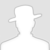FreeBuf.COM网络安全行业门户，每日发布专业的安全资讯、技术剖析。FreeBuf+小程序

2018-07-21 09:00:36

*本文中涉及到的相关漏洞已报送厂商并得到修复，本文仅限技术研究与讨论，严禁用于非法用途，否则产生的一切后果自行承担。

belluminarbank 是俄罗斯战队在 WCTF 上出的一道 EVM 题目，其中用到了很多 ETH 中经典的漏洞。虽然难度都不是很大，但是如果对 EVM 相关特性不了解的话还是有一定难度的，本文以这个题目为例详细介绍一下 ETH 智能合约中的安全性问题 。

``````pragma solidity ^0.4.23;contract BelluminarBank {
struct Investment {
uint256 amount;
uint256 deposit_term;
}
Investment[] balances;
bytes16 private secret;
function BelluminarBank(bytes16 _secret, uint256 deposit_term) public {
secret = _secret;
owner = msg.sender;
if(msg.value > 0) {
balances.push(Investment(msg.value, deposit_term, msg.sender));
}
}
function bankBalance() public view returns (uint256) {
}
function invest(uint256 account, uint256 deposit_term) public payable {
if (account >= head && account < balances.length) {
Investment storage investment = balances[account];
investment.amount += msg.value;
} else {
if(balances.length > 0) {
require(deposit_term >= balances[balances.length - 1].deposit_term + 1 years);
}
investment.amount = msg.value;
investment.deposit_term = deposit_term;
investment.owner = msg.sender;
balances.push(investment);
}
}
function withdraw(uint256 account) public {
require(now >= balances[account].deposit_term);
require(msg.sender == balances[account].owner);
msg.sender.transfer(balances[account].amount);
}
function confiscate(uint256 account, bytes16 _secret) public {
require(msg.sender == owner);
require(secret == _secret);
require(now >= balances[account].deposit_term + 1 years);

uint256 total = 0;
for (uint256 i = head; i <= account; i++) {
total += balances[i].amount;
delete balances[i];
}
msg.sender.transfer(total);
}
}``````

## 经典的 Integer OverFLow

EVM 使用的存储单位 uint256，即其中的栈、storage、memory 都是以 0x20 个字节为单位存储的，其可以表示的数据范围为 0 到 2^256 ,`0xFFFFFFFFFFFFFFFFFFFFFFFFFFFFFFFFFFFFFFFFFFFFFFFFFFFFFFFFFFFFFFFF` 可以看的其能够表示的范围是很大的，但是即使是这么大的范围依然存在溢出的可能性，当运算操作的结果大于 0x20 byte 所能表示的范围时，就会造成溢出导致判断失败。

1 years == 31536000

1 == 1 seconds

1 minutes == 60 seconds

1 hours == 60 minutes

1 days == 24 hours

1 weeks == 7 days

1 years == 365 days

## private 误区

``````0xc2575a0e9e593c00f959f8c92f12db2869c3395a3b0502d05e2516446f71f85b: Object
key: 0x0000000000000000000000000000000000000000000000000000000000000003
value: 0x41000000000000000000000000000000
``````

## 小心 Storage Pointer

``````0x405787fa12a823e0f2b7631cc41b3ba8828b3321ca811111fa75cd3aa3bb5ace: Object
key: 0x0000000000000000000000000000000000000000000000000000000000000002
``````

## 不可控的 this.balance

*本文作者FrogSec，转载请注明来自FreeBuf.COM

# 安全分析 # CTF # WCTF # 智能合约

+ 收入我的专辑
+ 加入我的收藏\
• 0 文章数
• 0 评论数
• 0 关注者